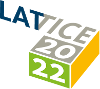#The 39th International Symposium on Lattice Field Theory (Lattice 2022)

Aug 8 – 13, 2022
Hörsaalzentrum Poppelsdorf
Europe/Berlin timezone

## Isothermal and isentropic speed of sound in (2+1)-flavor QCD at non-zero baryon chemical potential

Aug 11, 2022, 9:20 AM
20m
CP1-HSZ/0.011 (CP1-HSZ) - HS1 (CP1-HSZ)

### CP1-HSZ/0.011 (CP1-HSZ) - HS1

#### CP1-HSZ

450
Show room on map
Oral Presentation QCD at Non-zero Density

### Speaker

David Anthony Clarke (Bielefeld University)

### Description

Recently interest in calculations of the speed of sound in QCD under conditions like constant temperature $c_T^2$ or constant entropy per net baryon number $c_s^2$ arose in the discussion of experimental results coming from heavy ion experiments. It has been stressed that the former in particular is closely related to higher order cumulants of conserved charge fluctuations that are calculated in lattice QCD.

We present here results on $c_T^2$ and $c_s^2$ and compare results at vanishing strangeness chemical potential and vanishing net strangeness number with hadron resonance gas model calculations. We stress the difference of both observables at low temperature arising from the light meson sector, which does not contribute to $c_T^2$.

Using the scaling functions corresponding to the 3-$d$, O($N$) universality class, we discuss the imprint of the chiral transition on the speed of sound. Here we stress the energy-like behavior of net baryon-number fluctuations, which in the chiral limit has as a consequence that $c_T^2$ as well as $c_s^2$ will be sensitive to a peak in the specific heat that is characteristic for critical behavior of theories in 3-$d$, O($N$) spin models. Using data obtained on lattices with temporal extent $N_\tau=8$ and lighter-than-physical up and down quark masses, we conclude that this universal feature will show up in QCD only for very small values of the pion mass.

### Primary author

David Anthony Clarke (Bielefeld University)